# Mathematical Image Gallery

This is a gallery of images related to my mathematical research. I was inspired to create it because of the Illustrating Mathematics program at ICERM.

Click on the images to see larger versions.

## 2019:

### A curve in a quasi-periodic truchet tiling: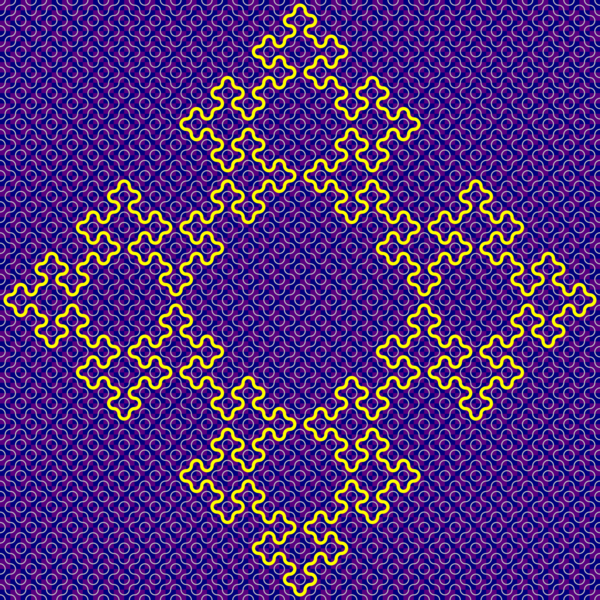This figure is built from Truchet tiles: square tiles with curves. There are two Truchet tiles, and a tiling is then determined by a map from the 2-dimensional integer lattice to a set of two elements. In this case the function chosen is quasi-periodic and defined in terms of the square root of 2. There is a natural sequence of closed curves surrounding the center which develop fractal symmetry in the limit as they get larger. One such curve is highlighted in yellow.

This is a remake of Figure 2 from the article "Renormalization of polygon exchange maps arising from corner percolation," Inventiones Mathematicae 191 (2013), Volume 191, no. 2, 255-320.

### The Necker cube surface: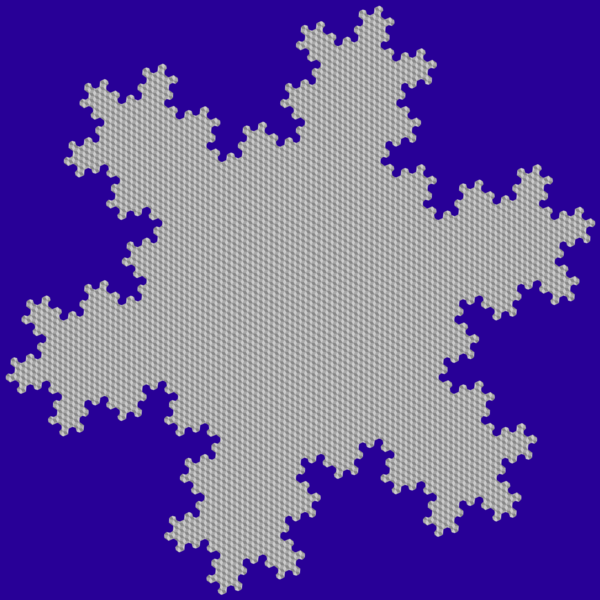The Necker cube surface is an infinite periodic surface built out of squares in space. If you look closely, you'll see the gray region is made out of cubes drawn in isometric projection. Here we have depicted a disk in the Necker cube surface with totally geodesic boundary.

The boundary of the disk approximates a fractal. Here the source of fractal symmetry comes from the slope of the boundary curve measured in a square it passes through. This slope is 239/169, a continued fraction approximate of the square root of 2.

This image and the related mathematical research is joint with Pavel Javornik. We hope to release preprints on the research soon.

### Refraction in the trihexagonal tiling: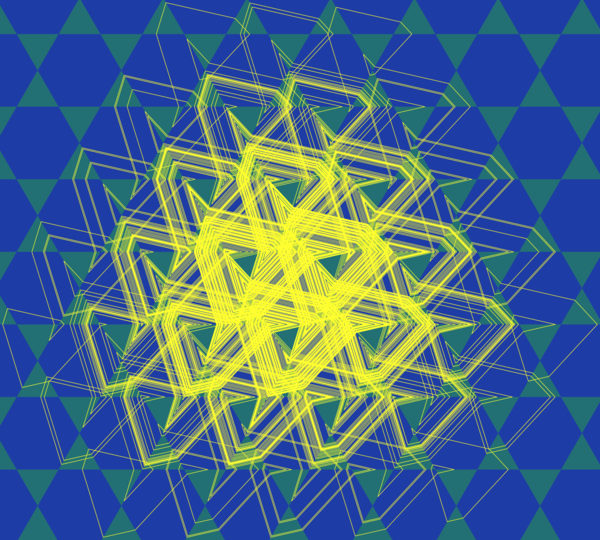In the trihexagonal tiling, regular triangles and hexagons meet edge to edge. Hexagons and triangles are made transparent materials of equal but opposite indices of refraction. In yellow we have drawn part of a ray of light refracting through the tiling. (Such a ray is also called a tiling billiard trajectory.) This trajectory starts with slope one and will densely fill a periodic subset of the plane, formed by removing a periodic family of triangles from the plane.

This figure and the related research is joint with Diana Davis. This is an alternate coloring of Figure 2 from "Periodicity and ergodicity in the trihexagonal tiling," Commentarii Mathematici Helvetici, 93 (2018) no. 4, 661--707.

### Rel deformations of the Arnoux-Yoccoz IET: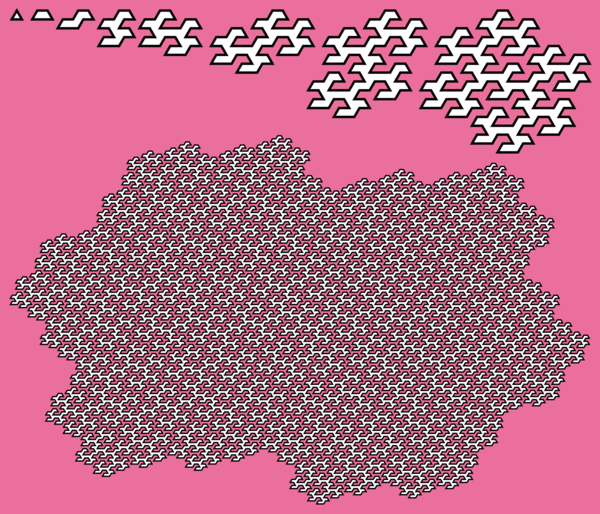The Arnoux-Yoccoz interval exchange transformation is self-renormalizable and hence ergodic. However all rel deformations of the IET are completely periodic. The arithmetic graph captures the algebraic dynamics of an IET, and periodic orbits correspond to loops in the graph. Depicted here are loops in the arithmetic graphs of rel deformations of the Arnoux-Yoccoz IET. The simplest loops are the largest deformations, and as the deformation amount tends to zero, the curves get more complicated, approaching a space filling curve.

This image and the associated research is joint with Barak Weiss. This is a different coloring of Figure 2 from "Rel leaves of the Arnoux-Yoccoz surfaces," Selecta Mathematica New Series (2018) 24, no. 2, 875-934.

### Conjugacy between an infinite IET and a rotation: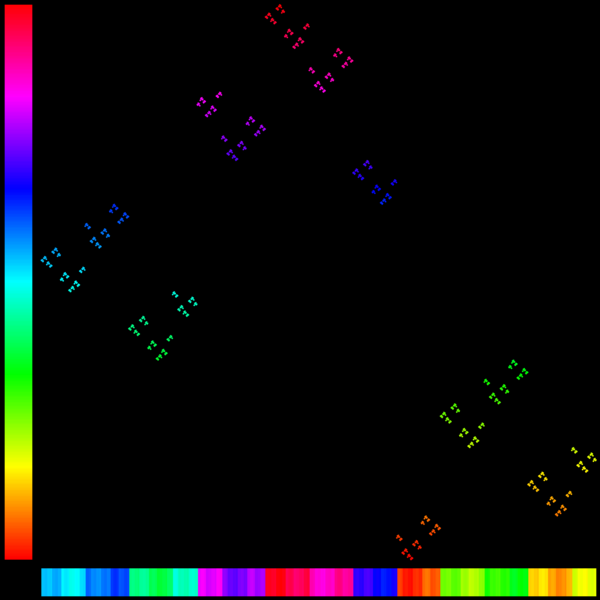The image depicts the graph of a measurable map from the interval to a circle. The measurable map conjugates the action of an infinite interval exchange transformation (IET) to the action of an irrational rotation. Both the IET and the rotation are defined over the field obtained by adjoining the square root of 17 to the rationals. The circle has a natural coloring by hue, and we pull it back to the interval (appearing as the x-axis). The graph admits some self similarity, you can find rescaled (and upside down) copies of the full graph appearing inside the graph.

This figure an the accompanying research is joint with Anna Tao. We hope to release a preprint soon.

HOME >>>>> GALLERY